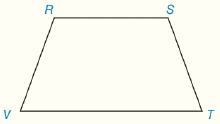Chapter 4.CT, Problem 12CTElementary Geometry For College St...

7th Edition
Alexander + 2 others
ISBN: 9781337614085

Solutions

Chapter
SectionElementary Geometry For College St...

7th Edition
Alexander + 2 others
ISBN: 9781337614085
Textbook Problem

In trapezoid RSTV, R S ¯ ∥ V T ¯ .a) Which sides are the legs of RSTV?b) Name two angles that are supplementary.To determine

To Find:

The legs of RSTV.

Explanation

Given In trapezoid RSTV, RS¯VT¯.

The two parallel sides of a trapezoid are called the base of a trapezoid and the other two sides are called legs of the trapezoid, from the given figure,

RS¯ and ST¯ are parallel sides and which is the base of the trapezoid and the other sides R

To determine

To Find:

The two angles that are supplementary.

Still sussing out bartleby?

Check out a sample textbook solution.

See a sample solution

The Solution to Your Study Problems

Bartleby provides explanations to thousands of textbook problems written by our experts, many with advanced degrees!

Get Started

Sketch the graphs of the equations in Exercises 512. 2y+x=1

Finite Mathematics and Applied Calculus (MindTap Course List)

What is the defining characteristic of a repeated-measures or within-subjects research design?

Essentials of Statistics for The Behavioral Sciences (MindTap Course List)

True or False: f(x) = 3x x3 is concave down for x 1.

Study Guide for Stewart's Single Variable Calculus: Early Transcendentals, 8th

True or False: The graph of x = 5 is a cylinder.

Study Guide for Stewart's Multivariable Calculus, 8th